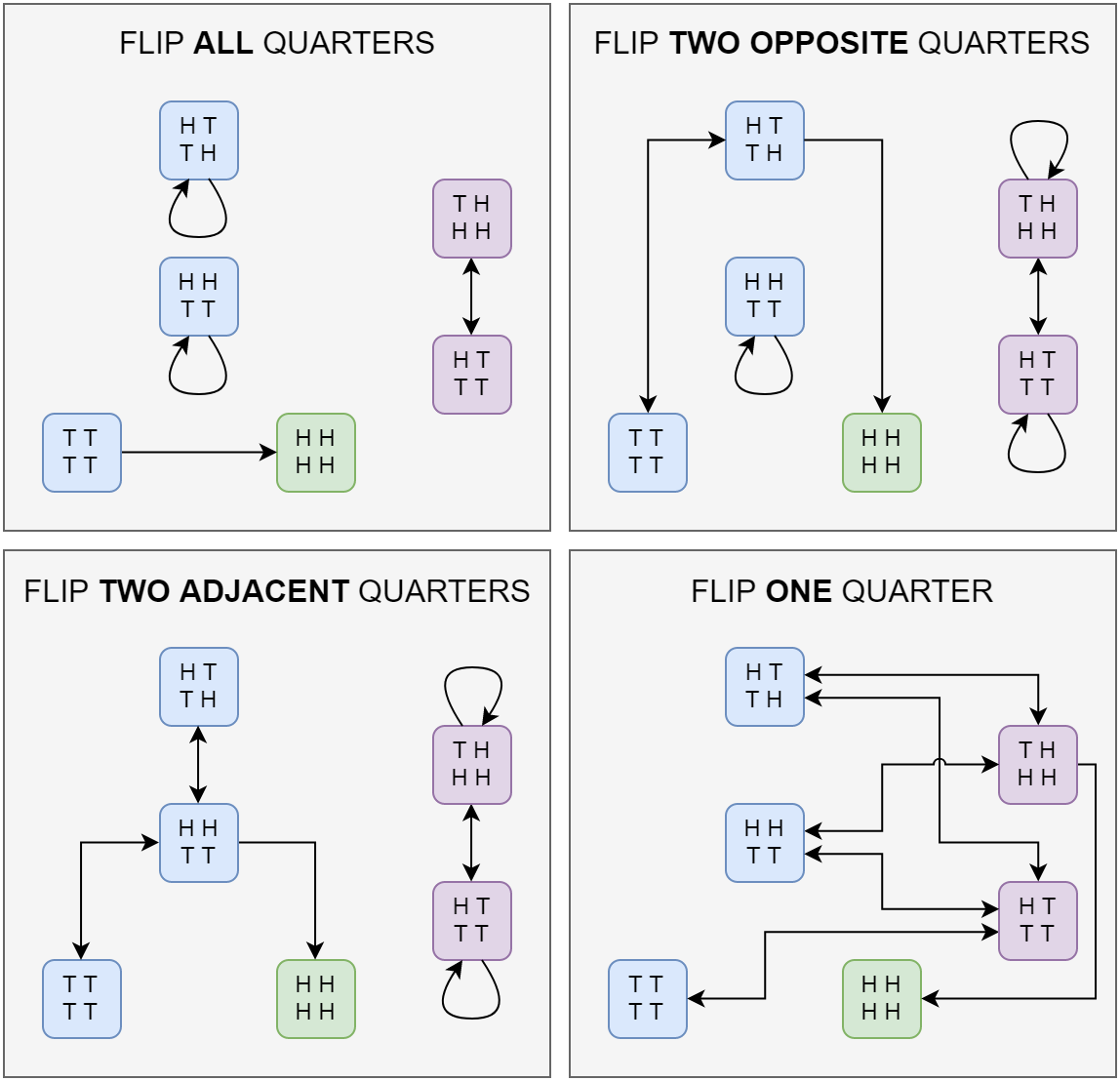# Quarter flipping on a spinning tableThis week’s Riddler Classic is a simple-sounding problem about statistics. But it’s not so simple! Here is a paraphrased version of the problem:

There is a square table with a quarter on each corner. The table is behind a curtain and thus out of your view. Your goal is to get all of the quarters to be heads up — if at any time all of the quarters are heads up, you will immediately be told and win.

The only way you can affect the quarters is to tell the person behind the curtain to flip over as many quarters as you would like and in the corners you specify. (For example, “Flip over the top left quarter and bottom right quarter,” or, “Flip over all of the quarters.”) Flipping over a quarter will always change it from heads to tails or tails to heads. However, after each command, the table is spun randomly to a new orientation (that you don’t know), and you must give another instruction before it is spun again.

Can you find a series of steps that guarantees you will have all of the quarters heads up in a finite number of moves?

Here is my solution:
[Show Solution]

## 11 thoughts on “Quarter flipping on a spinning table”

1.D says:

Cool. I suppose it depends a bit on what is meant by
“Can you find a series of steps that guarantees you will have all of the quarters heads up in a finite number of moves?”

If asserting that the number of moves is finite With Probability 1 is enough, I think a simple decision rule of at each position saying ‘flip kth coin’ and ‘do nothing’ (being n+1 distinct mutually exclusive choices selecting one at random, each with equal probability of $\frac{1}{n+1}$) is enough to guarantee an aperiodic, irreducible finite state (time homogenous) markov chain that includes all possible configurations. All states are visited WP1 and the time until first visit is stochastically smaller than some geometric random variable.

This is the random walk approach.

1.Laurent says:

Agreed.

My take was different: I interpreted the question to mean that the algorithm had to have a uniform bound on stopping time that was robust to adversarial table spins. So really, I assumed the table spins weren’t random at all.

2.Michael Branicky says:

Laurent, Nice solution!

I modeled as a non-deterministic finite automaton that tracks all the possibilities (or all the possibilities with non-zero probability wrt the previous post). Then, I used breadth-first search on it to find the optimal 15-move solution.

Write up and code here:

1.Alex Matulich says:

I did some statistical analysis over several hundred trials and found that the distribution of the number of steps required to reach a solution is pretty flat. I thought there might be an expectancy of some likely number of steps (a bell curve) but that wasn’t the case.

2.Austin Aslett says:

15 moves maximum.

A4 = flip all 4 coins
BR= flip bottom right coin
TLBR= flip top left, bottom right coins
B2= flip bottom 2 coins

I think the following sequence of steps will win every time:

A4
BR
A4
TLBR
A4
B2
A4
TLBR
A4
BR
A4
B2
A4
TLBR
A4

3.Kurt Smith says:

Nice illustration of the state diagram!
To be pedantic, it’s probably more accurate to say this problem is about probability (or combinatorics) than statistics 😉

4.Joshua Meisel says:

I solved for n=2^k coins, using a recursive trick where you simultaneously play two games half the size. Your solution matches the recursive solution.

https://joshuameisel.github.io/Spinning-Table-Riddle/

It also turns out there’s no solution for any other n.

5.Charlie says:

You listed 5 possible moves, and it seems 1 of them (TRI) was omitted from the discussion. Can you explain why?

1.Laurent says:

It turns out this move is not needed. If you work out the transition diagram for (TRI) as I did with the other moves, you’ll find that it is very similar to the diagram for (ONE). In fact, you can take the solution I found and replace ONE with TRI and it still works!

1.Dovi Lilling says:

I’d say the reason is because (TRI) is equivalent to (ONE) + (ALL) move, and (ALL) does not really change the state (except TTTT -> HHHH, which finishes the game).

6.Sayan Dasgupta says:

you need 7 moves at maximum by switching between diagonals and sides. also note that out of 16 possible combinations, there are 8 pairs of equivalent classes like HHHH and TTTT. Since the starting position is not one of the above, so only 7 moves (at max) are needed to get to a solution.